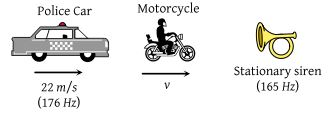The difference between the apparent frequency of a source of sound as perceived by an observer during its approach and recession is 2% of the natural frequency of the source. If the velocity of sound in air is 300 m/sec, the velocity of the source is : (It is given that velocity of source << velocity of sound)

(1) 6 m/sec

(2) 3 m/sec

(3) 1.5 m/sec

(4) 12 m/sec

Concept Questions :-

Doppler effect
High Yielding Test Series + Question Bank - NEET 2020

Difficulty Level:

Two whistles A and B produce notes of frequencies 660 Hz and 596 Hz respectively. There is a listener at the mid-point of the line joining them. Now the whistle B and the listener start moving with speed 30 m/s away from the whistle A. If the speed of sound be 330 m/s, how many beats will be heard by the listener :

(1) 2

(2) 4

(3) 6

(4) 8

Concept Questions :-

Beats
High Yielding Test Series + Question Bank - NEET 2020

Difficulty Level:

A source producing the sound of frequency 170 Hz is approaching a stationary observer with a velocity of 17 ms–1. The apparent change in the wavelength of sound heard by the observer is (speed of sound in air = 340 ms–1

(1) 0.1m

(2) 0.2m

(3) 0.4m

(4) 0.5m

Concept Questions :-

Doppler effect
High Yielding Test Series + Question Bank - NEET 2020

Difficulty Level:

A police car moving at 22 m/s, chases a motorcyclist. The policeman sounds his horn at 176 Hz, while both of them move towards a stationary siren of frequency 165 Hz. Calculate the speed of the motorcycle, if it is given that he does not observe any beats(1) 33 m/s

(2) 22 m/s

(3) Zero

(4) 11 m/s

Concept Questions :-

Beats
High Yielding Test Series + Question Bank - NEET 2020

Difficulty Level:

An observer moves towards a stationary source of sound with a speed 1/5th of the speed of sound. The wavelength and frequency of the sound emitted are λ and f respectively. The apparent frequency and wavelength recorded by the observer are respectively :

1.

2. $f,\text{\hspace{0.17em}}1.2\lambda$

3. $0.8f,\text{\hspace{0.17em}}0.8\lambda$

4. $1.2f,\text{\hspace{0.17em}}1.2\lambda$

Concept Questions :-

Standing waves
High Yielding Test Series + Question Bank - NEET 2020

Difficulty Level:

The equation of displacement of two waves are given as ${y}_{1}=10\mathrm{sin}\left(3\pi t+\frac{\pi }{3}\right)$; ${y}_{2}=5\left(\mathrm{sin}3\pi t+\sqrt{3}\mathrm{cos}3\pi t\right)$. Then what is the ratio of their amplitudes ?

1. 1 : 2

2. 2 : 1

3. 1 : 1

4. None of these

Concept Questions :-

Wave motion
High Yielding Test Series + Question Bank - NEET 2020

Difficulty Level:

Consider ten identical sources of sound all giving the same frequency but having phase angles which are random. If the average intensity of each source is I0, the average of resultant intensity I due to all these ten sources will be :

(1) I = 100 I0

(2) I = 10 I0

(3) I = I0

(4) $I=\sqrt{10}\text{\hspace{0.17em}}{I}_{0}$

Concept Questions :-

Energy of waves
High Yielding Test Series + Question Bank - NEET 2020

Difficulty Level:

41 forks are so arranged that each produces 5 beats per sec when sounded with its near fork. If the frequency of the last fork is double the frequency of the first fork, then the frequencies of the first and last fork are respectively :

(1) 200, 400

(2) 205, 410

(3) 195, 390

(4) 100, 200

Concept Questions :-

Beats
High Yielding Test Series + Question Bank - NEET 2020

Difficulty Level:

Two identical wires have the same fundamental frequency of 400 Hz when kept under the same tension. If the tension in one wire is increased by 2%, the number of beats produced will be :

1. 4

2. 2

3. 8

4. 1

Concept Questions :-

Beats
High Yielding Test Series + Question Bank - NEET 2020

Difficulty Level:

16 tunning forks are arranged in the order of increasing frequencies. Any two successive forks give 8 beats per sec when sounded together. If the frequency of the last fork is twice the first, then the frequency of the first fork is

(1) 120

(2) 160

(3) 180

(4) 220

Concept Questions :-

Standing waves